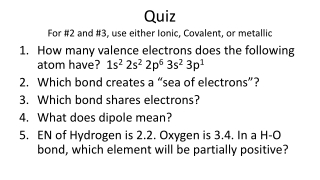Download PresentationQuiz For #2 and #3, use either Ionic, Covalent, or metallic

# Quiz For #2 and #3, use either Ionic, Covalent, or metallic

Télécharger la présentation## Quiz For #2 and #3, use either Ionic, Covalent, or metallic

- - - - - - - - - - - - - - - - - - - - - - - - - - - E N D - - - - - - - - - - - - - - - - - - - - - - - - - - -
##### Presentation Transcript

1. Quiz For #2 and #3, use either Ionic, Covalent, or metallic • How many valence electrons does the following atom have? 1s2 2s2 2p6 3s2 3p1 • Which bond creates a “sea of electrons”? • Which bond shares electrons? • What does dipole mean? • EN of Hydrogen is 2.2. Oxygen is 3.4. In a H-O bond, which element will be partially positive?

2. 8.2 Ionic Compounds

3. What is the difference between ionic and covalent bonds? • What is the difference between polar and non-polar covalent bonds? • Why are electrons shared equally or unequally?

4. Are bonds ever pure? • No! The bonds are measured in a gradient. • As the difference between the electronegativity (EN) increases, the bonds move from non-polar covalent to polar covalent to ionic

5. If the difference in EN is 2.0 or greater, ionic • If difference is 0.5 or greater, polar covalent bond • If difference is anything less than 0.5 is non-polar covalent

6. Percent Ionic Character • The percentage of the ionic character of a bond can be calculated using the following equation: XA is the larger EN of the two elements XA XB

7. What is the percent ionic character between silicon and fluorine? • EN of Si= 1.8 • EN of F= 4.0 • = 55% ionic

8. Identify whether the following bonds are non-polar covalent, polar covalent, or ionic. Also calculate the % ionic character. • HF • KCl • HBr • CH4 • Even though there are 4 H, do not quadruple the EN. You are calculating the difference of EN between a single carbon and hydrogen • Br3N

9. Polyatomic ions • Polyatomic ions can have covalent bonds within the ion, but will bond ionically with other ions • The nitrogen and oxygen in NO3- are bonded covalently, but due to an extra electron, the compound becomes charged

10. Electron-dot structure, or Lewis Structure • In a Lewis structure, dots represents the valence electrons an atom has • It can also portray covalent bonds between two atoms H Cl HCl Shared electrons

11. Generally, you will fill in the dots in the following numbered order around the element 3 6 A 4 7 1 2 5 8

12. Draw the Lewis structure • Li • Mg • Al • As • Br • Ne

13. Lewis structure for Ionic compound • Draw the Lewis structure for K+ • It is just drawn as K+ because it has lost an electron • Draw the Lewis structure for Br- • It is drawn as [ Br ] – • Brackets are added for all anions (negative ions) • Make sure to place the charge outside of brackets • The ionic compound KBr can be written as: [ Br ] – Br K K+

14. Draw the Lewis structure for MgCl2 Mg2+ [ Cl ]2- • You do not need to draw out two Cl atoms, just place the subscript outside of the brackets • Draw the Lewis structure for Na2S Na2+ [ S ]2-

15. Draw the Lewis structure for Al2S3 Al23+[ S ]32-

16. [ Cl ]22– [ Cl ]2– Be2+ Be2+ c. a. [ Cl ]2– Be2 [ Cl ] 2– d. Be2+ b.

17. P P c. P P a. P 2 P P d. b.